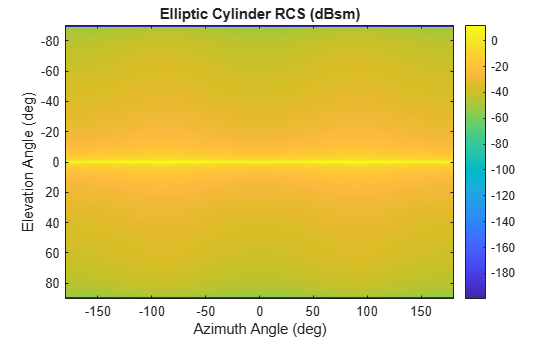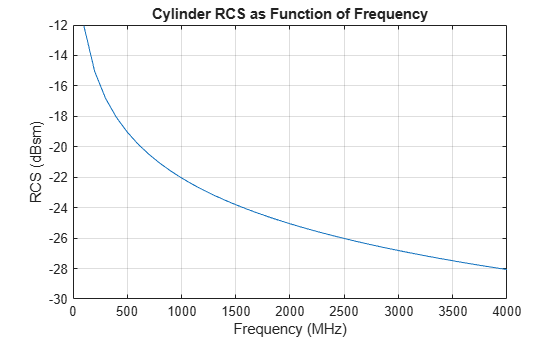# rcscylinder

## Syntax

``rcspat = rcscylinder(r1,r2,height,c,fc)``
``rcspat = rcscylinder(r1,r2,height,c,fc,az,el)``
``[rcspat,azout,elout] = rcscylinder(___)``

## Description

example

````rcspat = rcscylinder(r1,r2,height,c,fc)` returns the radar cross section pattern of an elliptical cylinder having a semi-major axis, `r1`, a semi-minor axis, `r2`, and a height, `height`. The radar cross section is a function of signal frequency, `fc`, and signal propagation speed,`c`. The bottom of the cylinder lies on the xy-plane. The height of the cylinder points along the positive z-axis.```

example

````rcspat = rcscylinder(r1,r2,height,c,fc,az,el)` also specifies the azimuth angles, `az`, and elevation angles, `el`, at which to compute the radar cross section.```

example

````[rcspat,azout,elout] = rcscylinder(___)` also returns the azimuth angles, `azout`, and elevation angles, `elout`, at which the radar cross sections are computed. You can use these output arguments with any of the previous syntaxes.```

## Examples

collapse all

Display the radar cross section (RCS) pattern as a function of azimuth and elevation for an elliptical cylinder whose semi-major axis is 12.5 cm and whose semi-minor axis is 9 cm. The cylinder height is 1 m. The operating frequency is 4.5 GHz.

Specify the cylinder geometry and signal parameters.

```c = physconst('Lightspeed'); fc = 4.5e9; rada = 0.125; radb = 0.090; hgt = 1;```

Compute the RCS for all directions using the default direction values.

```[rcspat,azresp,elresp] = rcscylinder(rada,radb,hgt,c,fc); imagesc(azresp,elresp,pow2db(rcspat)) colorbar xlabel('Azimuth Angle (deg)') ylabel('Elevation Angle (deg)') title('Elliptic Cylinder RCS (dBsm)')```Plot the radar cross section (RCS) pattern of an elliptical cylinder as a function of elevation at a constant azimuth angle of 5${}^{\circ }$. The cylinder has a semi-major axis of 12.5 cm and a semi-minor axis of 9 cm. The cylinder height is 1 m. The operating frequency is 4.5 GHz.

Specify the cylinder geometry and signal parameters.

```c = physconst('Lightspeed'); fc = 4.5e9; rada = 0.125; radb = 0.090; hgt = 1;```

Compute the RCS for all elevation angles at a fixed azimuth angle of 5${}^{\circ }$.

```el = -90:90; az = 5; [rcspat,azresp,elresp] = rcscylinder(rada,radb,hgt,c,fc,az,el); plot(elresp,pow2db(rcspat)) xlabel('Elevation Angle (deg)') ylabel('RCS (dBsm)') title('Elliptic Cylinder RCS as Function of Elevation') grid on```Plot the radar cross section (RCS) of an elliptical cylinder as a function of frequency for a fixed direction. The cylinder has as semi-major axis of 12.5 cm and a semi-minor axis of 9 cm. The cylinder height is 1 m.

Specify the cylinder geometry and signal parameters.

```c = physconst('Lightspeed'); rada = 0.125; radb = 0.090; hgt = 1;```

Compute radar cross sections as a function of frequency for a fixed azimuth and elevation.

```az = 5.0; el = 20.0; fc = (100:100:4000)*1e6; [rcspat,azpat,elpat] = rcscylinder(rada,radb,hgt,c,fc,az,el); disp([azpat,elpat])```
``` 5 20 ```
```plot(fc/1e6,pow2db(squeeze(rcspat))) xlabel('Frequency (MHz)') ylabel('RCS (dBsm)') title('Cylinder RCS as Function of Frequency') grid on```## Input Arguments

collapse all

Length of semi-major axis of cylinder, specified as a positive scalar. Units are in meters.

Example: `5.5`

Data Types: `double`

Length of semi-minor axis of cylinder, specified as a positive scalar. Units are in meters.

Example: `3.0`

Data Types: `double`

Height of cylinder, specified as a positive scalar. Units are in meters.

Example: `3.0`

Data Types: `double`

Signal propagation speed, specified as a positive scalar. Units are in meters per second. For the SI value of the speed of light, use `physconst('LightSpeed')`.

Example: `3e8`

Data Types: `double`

Frequency for computing radar cross section, specified as a positive scalar or positive, real-valued, 1-by-L row vector. Frequency units are in Hz.

Example: `[100e6 200e6]`

Data Types: `double`

Azimuth angles for computing directivity and pattern, specified as a real-valued 1-by-M row vector where M is the number of azimuth angles. Angle units are in degrees. Azimuth angles must lie between –180° and 180°, inclusive.

The azimuth angle is the angle between the x-axis and the projection of a direction vector onto the xy-plane. The azimuth angle is positive when measured from the x-axis toward the y-axis.

Example: `-45:2:45`

Data Types: `double`

Elevation angles for computing directivity and pattern, specified as a real-valued, 1-by-N row vector where N is the number of desired elevation directions. Angle units are in degrees. Elevation angles must lie between –90° and 90°, inclusive.

The elevation angle is the angle between a direction vector and xy-plane. The elevation angle is positive when measured towards the z-axis.

Example: `-75:1:70`

Data Types: `double`

Tip

To construct a circular cylinder, set `r2` equal to `r1`.

## Output Arguments

collapse all

Radar cross section pattern, returned as a real-valued N-by-M-by-L array. N is the length of the vector returned in the `elout` argument. M is the length of the vector returned in the `azout` argument. L is the length of the `fc` vector. Units are in meters-squared.

Data Types: `double`

Azimuth angles for computing directivity and pattern, returned as a real-valued 1-by-M row vector where M is the number of azimuth angles specified by the `az` input argument. Angle units are in degrees.

The azimuth angle is the angle between the x-axis and the projection of the direction vector onto the xy-plane. The azimuth angle is positive when measured from the x-axis toward the y-axis.

Data Types: `double`

Elevation angles for computing directivity and pattern, returned as a real-valued 1-by-N row vector where N is the number of elevation angles specified in `el` output argument. Angle units are in degrees.

The elevation angle is the angle between the direction vector and xy-plane. The elevation angle is positive when measured towards the z-axis.

Data Types: `double`

collapse all

### Azimuth and Elevation

This section describes the convention used to define azimuth and elevation angles.

The azimuth angle of a vector is the angle between the x-axis and its orthogonal projection onto the xy-plane. The angle is positive when going from the x-axis toward the y-axis. Azimuth angles lie between –180° and 180° degrees, inclusive. The elevation angle is the angle between the vector and its orthogonal projection onto the xy-plane. The angle is positive when going toward the positive z-axis from the xy-plane. Elevation angles lie between –90° and 90° degrees, inclusive.Mahafza, Bassem. Radar Systems Analysis and Design Using MATLAB, 2nd Ed. Boca Raton, FL: Chapman & Hall/CRC, 2005.